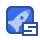# matlab泊松分布验证代码-Probability_Distributions_Comparisons:使用MATLAB2020a验证各种近30收藏

matlab泊松分布验证代码概率分布比较 该项目是B.Tech三年级概率和随机过程课程的一部分，在该课程中，我试图验证以下近似值并绘制不同概率分布的概率分布函数/概率质量函数以进行比较。 二项分布趋于正态分布 二项分布趋于泊松分布 泊松分布趋于正态分布 趋于二项式分布的超几何分布 该项目是使用MATLAB 2020a完成的。 存储库内容：- binomial_and_normal.m-用于验证二项分布趋于正态分布的近似值的MATLAB代码 binomial_and_poisson.m-用于验证二项分布趋于泊松分布的近似值的MATLAB代码 poisson_and_normal.m-用于验证Poisson分布趋于正态分布的近似值的MATLAB代码 hypergeometric_and_binomial.m-用于验证超几何分布趋于二项分布的近似值的MATLAB代码 binomial_vs_normal.pdf-包含用于验证案例的代码和图的PDF文件 binomial_vs_poisson.pdf-包含用于验证案例的代码和图的PDF文件 poisson_vs_normal.pdf-包含用于验

...展开详情

• 943
资源
• 3
粉丝
•等级
•至尊王者matlab泊松分布验证代码-Probability_Distributions_Comparisons:使用MATLAB2020a验证各种近 40积分/C币 立即下载
1/0